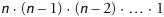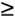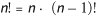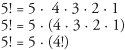# Example Using Recursion: Factorials

### Example Using Recursion Factorials

Let us write a recursive program to perform a popular mathematical calculation. Consider the factorial of a positive integer n, written n! (and pronounced "n factorial"), which is the productwith 1! equal to 1 and 0! defined to be 1. For example, 5! is the product 5 · 4 · 3 · 2 · 1, which is equal to 120.

The factorial of integer number (where number0) can be calculated

``` factorial = 1;
for ( int counter = number; counter >= 1; counter-- )
factorial *= counter;
```

A recursive declaration of the factorial method is arrived at by observing the following relationship:For example, 5! is clearly equal to 5 · 4!, as is shown by the following equations:The evaluation of 5! would proceed as shown in Fig. 15.2. Figure 15.2(a) shows how the succession of recursive calls proceeds until 1! (the base case) is evaluated to be 1, which terminates the recursion. Figure 15.2(b) shows the values returned from each recursive call to its caller until the final value is calculated and returned.

Figure 15.2. Recursive evaluation of 5!.

(This item is displayed on page 748 in the print version)Figure 15.3 uses recursion to calculate and print the factorials of the integers from 010. The recursive method factorial (lines 713) first tests to determine whether a terminating condition (line 9) is TRue. If number is less than or equal to 1 (the base case), factorial returns 1, no further recursion is necessary and the method returns. If number is greater than 1, line 12 expresses the problem as the product of number and a recursive call to factorial evaluating the factorial of number - 1, which is a slightly simpler problem than the original calculation, factorial( number ).

Figure 15.3. Factorial calculations with a recursive method.

 ``` 1 // Fig. 15.3: FactorialCalculator.java 2 // Recursive factorial method. 3 4 public class FactorialCalculator 5 { 6 // recursive method factorial 7 public long factorial( long number ) 8 { 9 if ( number <= 1 ) // test for base case 10 return 1; // base cases: 0! = 1 and 1! = 1 11 else // recursion step 12 return number * factorial( number - 1 ); 13 } // end method factorial 14 15 // output factorials for values 0-10 16 public void displayFactorials() 17 { 18 // calculate the factorials of 0 through 10 19 for ( int counter = 0; counter <= 10; counter++ ) 20 System.out.printf( "%d! = %d ", counter, factorial( counter ) ); 21 } // end method displayFactorials 22 } // end class FactorialCalculator ```

Common Programming Error 15.1Either omitting the base case or writing the recursion step incorrectly so that it does not converge on the base case can cause a logic error known as infinite recursion, where recursive calls are continuously made until memory has been exhausted. This error is analogous to the problem of an infinite loop in an iterative (nonrecursive) solution.

Method displayFactorials (lines 1621) displays the factorials of 010. The call to method factorial occurs in line 20. Method factorial receives a parameter of type long and returns a result of type long. Figure 15.4 tests our factorial and displayFactorials methods by calling displayFactorials (line 10). As can be seen from the output of Fig. 15.4, factorial values become large quickly. We use type long (which can represent relatively large integers) so the program can calculate factorials greater than 12!. Unfortunately, the factorial method produces large values so quickly that factorial values soon exceed the maximum value that can be stored even in a long variable.

Figure 15.4. Testing the factorial method.

``` 1 // Fig. 15.4: FactorialTest.java
2 // Testing the recursive factorial method.
3
4 public class FactorialTest
5 {
6 // calculate factorials of 0-10
7 public static void main( String args[] )
8 {
9 FactorialCalculator factorialCalculator = new FactorialCalculator();
10 factorialCalculator.displayFactorials();
11 } // end main
12 } // end class FactorialTest
```

 ```0! = 1 1! = 1 2! = 2 3! = 6 4! = 24 5! = 120 6! = 720 7! = 5040 8! = 40320 9! = 362880 10! = 3628800 ```

Due to the limitations of integral types, float or double variables may ultimately be needed to calculate factorials of larger numbers. This points to a weakness in most programming languagesnamely, that the languages are not easily extended to handle the unique application requirements. As we saw in Chapter 9, Java is an extensible language that allows us to create arbitrarily large integers if we wish. In fact, package java.math provides classes BigInteger and BigDecimal explicitly for arbitrary precision mathematical calculations that cannot be performed with primitive types. For more information on these classes visit, java.sun.com/j2se/5.0/docs/api/java/math/BigInteger.html and java.sun.com/j2se/5.0/docs/api/java/math/BigDecimal.html, respectively.Java How to Program (6th Edition) (How to Program (Deitel))
ISBN: 0131483986
EAN: 2147483647
Year: 2003
Pages: 615

Similar book on Amazon